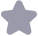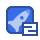### 算法竞赛入门经典各章习题答案收藏 20

int main Int n double a scanf("%od",&n) a=n*95.0 if(a<300) printf("%.2If\n", a else printf( %.2If\n, a*0.85) return u 题1 1-8 Include stdio h> Include math. h> int main() double n. scanf("‰f"&n} printf(%.2If",fabs(n) return 0: 习题1 #include <stdio. h> int main( int a, b scanf("‰d%‰d%d",&a,&b,&c); printf("no\n") if(a’a+bbc)||(aa+c=-b+b川|b*b+C*c==aa) printf("yes\n") else print("no\n return 0. 习题1-10 Include <stdio. h int main int n: f("%d",&n if(n‰4=0) fn%100l=0) printf("no\n") se if(n%400==0) printf("yes, n"); tf("non") else fl"no\n"y return 0: 第二章 习题2-1 Include <stdio. h> int main() int n, count=0 scanf("‰d",&n} while(n>0) count++ n=n/10 printf("%d\n"count): return o 习题2-2 Include <stdio.h> int main() int a, b for(inti=100<=999++) a=%10; b=i/10%10 c=i/100 b*b+c*c*c】 printf%d\n i) return o 习题2-3 Include <stdio. h> int main() i a b c sar("‰d%d%d,&a&b&C; for(=10j<=100|++) f(%3==&&%5-=b&&%7=cC print( %\"); if(i==101) printf("no answer\n") return o 习题24 Include <stdio. h> int main() i,j, k, h scant("%od, &n for(i-n; i>O; i-) for(k=0; k<n-i; k++) printf( for(j=0j<2*ⅰ-1j+) printf("#") f"n"); return o 习题2-5 文件题,南邮竞赛基本不涉及。 习题2-6 Include <stdio. h> int main L n double sum=1.0 scanf("‰d",&n} for(=2;<=n;i+ sum+=(10/); printf("9.3If\n",sum) return 0 习题2-7 Include stdio h> Include <math. h> int main() int t=-1 double a=.0. sum=1.0 whie(fabs(a)>=0.000001} a=1/(a+2) a== sum=sum+a t=t(-1) printf( %.9If\n",sum) return 0. 习题2-8 Include <stdio. h> int main( int i, n, m, temp; double sum=0 sanf("‰d%d",&n&m); if(n>m) temp=n; n=m m=temp for(i=n; i<=m; i++) sum=sum+(1.0/i/ printf( 9.5If\n ,sum return o 习题2-9 printf的特殊用法:对于m.n的格式可以用如下方法表示 char ch printf(%∵sin",m,nch) 前边的定义的是总的宽度,后边的定义的是输出的个数。分别对应外面的参数m和n。这 种方法的好处是可以在语句之外对参数m和n赋值,从而控制输出格式。 Include <stdio. h In, mal int a, b, c scanf("%dd%d, &a, &b, &c) printf (%.*If\n",,(double)a/b) return 习题2-10 Hinc lude <stdio. h> int main( int. a, b, c, d, e, t, g, h for(b=l; b<=y; bI 1) for(c=1;c<=9:c+) for(d=1;d<=9;d++) for(e=1;a<=9;e++ fnr(f=1;f<=9;f-+) for(g=1:g<=9;g++) for(h=1;h<=9;h++) if((2水(a*100+*10c)==1水(100e10-f)Rk(3(a*100+b*10c)==1*(g*100h*10+i) R(a!-b)&(a!-C)&(a!-d)(a!-日)&(a!-)&&(a!-g)&(a!-h)&(a!-i)(b!c)&&(b!-d)&( !-c)&(b!-f)&(b!g)&&(b!-h)&(!-1&(c!一小题(c!c)&(c!一f)(e!g)&k(c!-h)&(c!-i )&&(d!=e)&(d!=)kk(d!g)k&(d!h)&(d!=1)&(e!=f)k(e!=g)&(e!=h)&(e!=i)&k(!=g)kk (f!=h)&&(!=i)(g!=h)&(s!=)&(h!=)) printf("%d,%,%n”,a*100+3*1(+C,小*100+e*10+,g*100+h*10-i); return (: 第二三章 习题3-1 finc lude <stdio. h include <string. h int main o int i, a[101, n, max: memset(a, sizeof(a), D) while(scanr(%ou", &n==1) aX-日 or(i=1;i<101;i++) f(ail>=max max-ali] for(i=0;i<101;i++) f(ali]==max print十 n); 习题3-2 Include <stdio. hs Finc lude 'ctype. h> char s1000]

...展开详情

一个资源只可评论一次，评论内容不能少于5个字Paul_Guderian 我想看的是DP一章的答案，结果收到了1-3章的答案。深深的欺骗，无言以对。
2017-01-26
回复
2016-04-11
回复
2015-10-20
回复
2015-10-10
回复单片机学徒 虽然不是很完美，但是帮助挺大的，比自己做一题想老半天强多了。
2015-09-06
回复
2015-08-14
回复
2015-08-14
回复
2015-07-04
回复7bae 很不错，有点可惜是不是很全，但是仍然很感谢楼主分享！
2015-05-24
回复
2015-04-12
回复
tuqinag关注 私信 TA的资源
上传资源赚积分,得勋章
最新推荐算法竞赛入门经典各章习题答案 32积分/C币 立即下载
1/15试读已结束，剩余10页未读...

32积分/C币 立即下载 ＞